### KarthikYearning Deep Learning Writing Project Dataset Web About Contact

This blog provides indepth understanding of optimization from Introduction to deep learning course by CMU .

Course Page

In neural networks, the convergence is attained by finding the optimal network error. The error is defined as a function called the Loss function, which must be minimized through an iterative process of weight updates w.r.t the gradients.

Gradients are defined as the rate of change of Loss function w.r.t the multivariate weights. The optimization problem is complicated since neural network learns a non-linear function to understand the training data.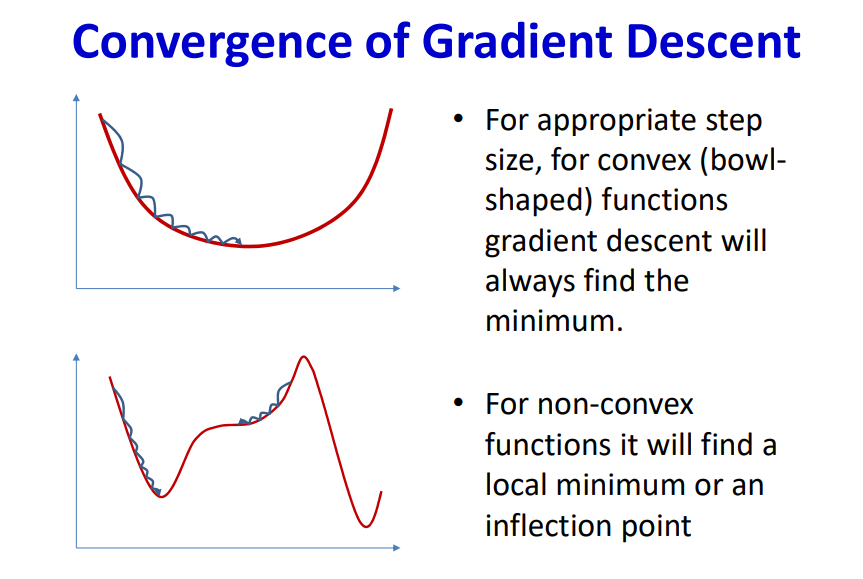### The problem of Optimization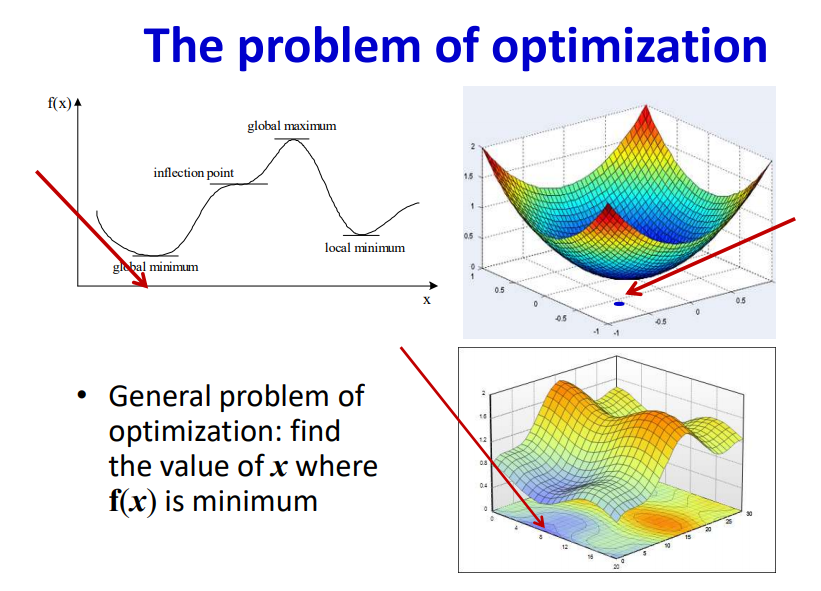The minimum value of Loss function facilitates the network with convergence. But in general, the loss function is a non-convex function which makes the process of finding the lowest point complicated.

By employing gradient of the multivariate function we can navigate to the minimum point. There are possibilities that the local minimum can be misunderstood as global minimum during the optimization process.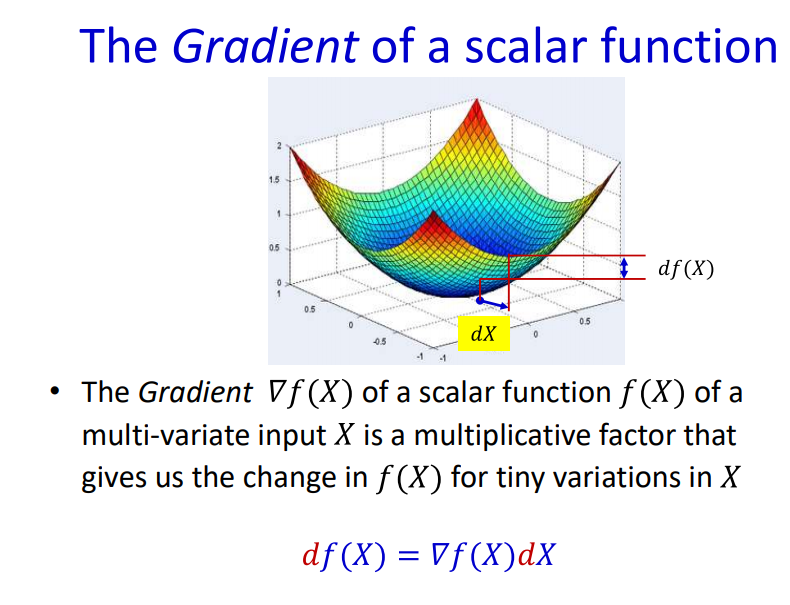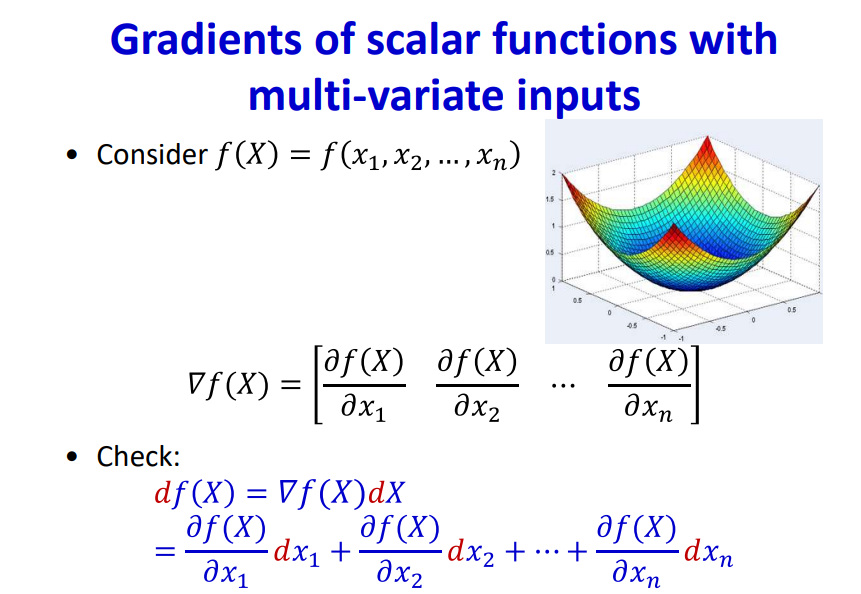The rate of change of Loss function w.r.t all the weights in the network provides a comprehensive dependency of network weights in minimizing the loss.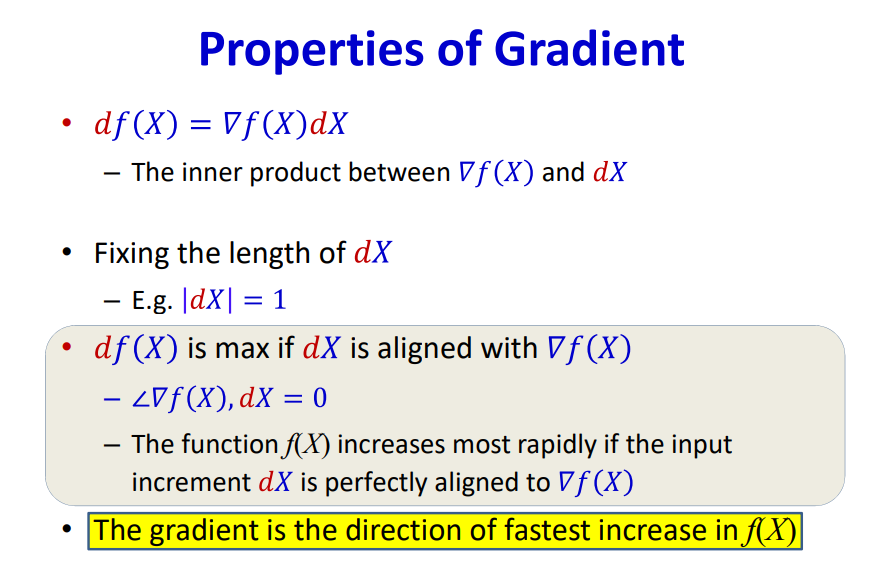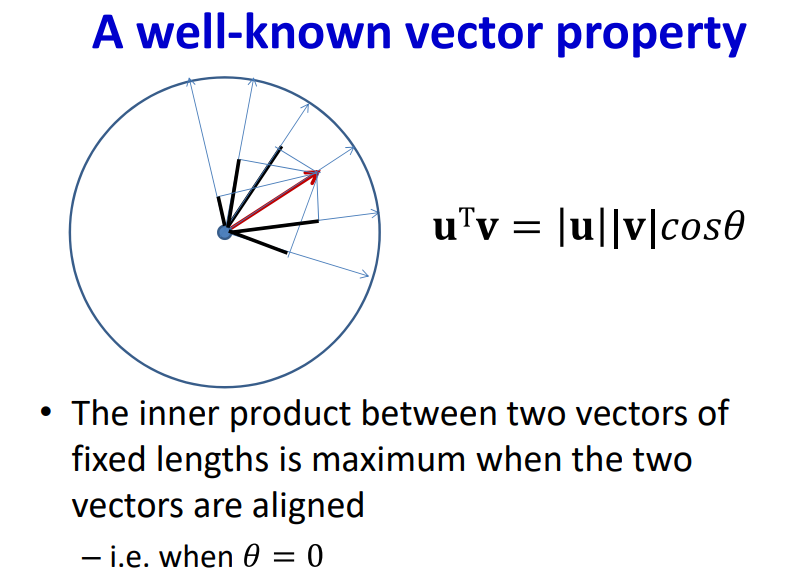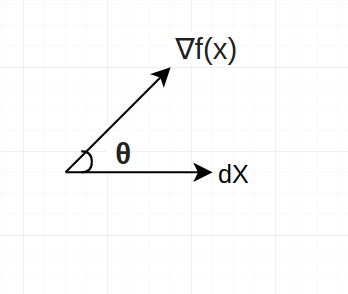When both the vector are aligned i.e when the value of θ = 0 and Cos(θ) = 1. Hence the both vectors must be aligned to move in the fastest changing diection. The direction is obtained by the gradient sign.

The sign plays the major role in navigating towards the minimum/maximum loss point in the non-convex loss function.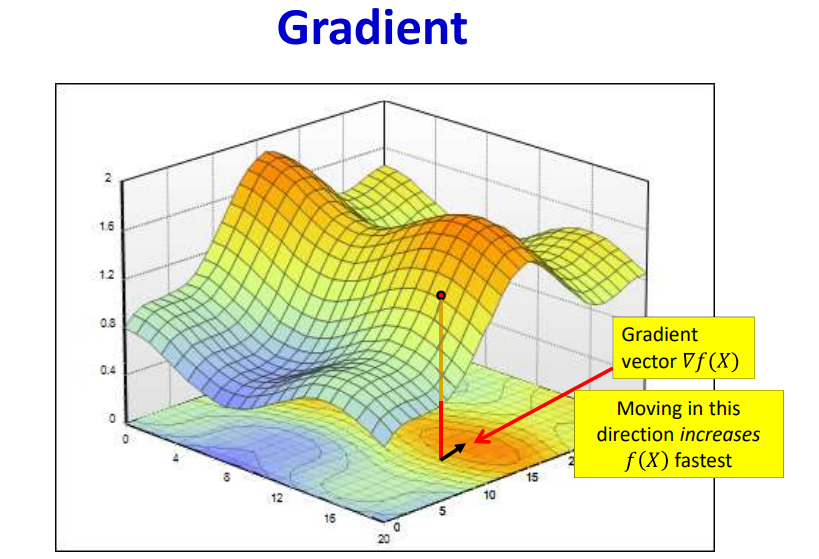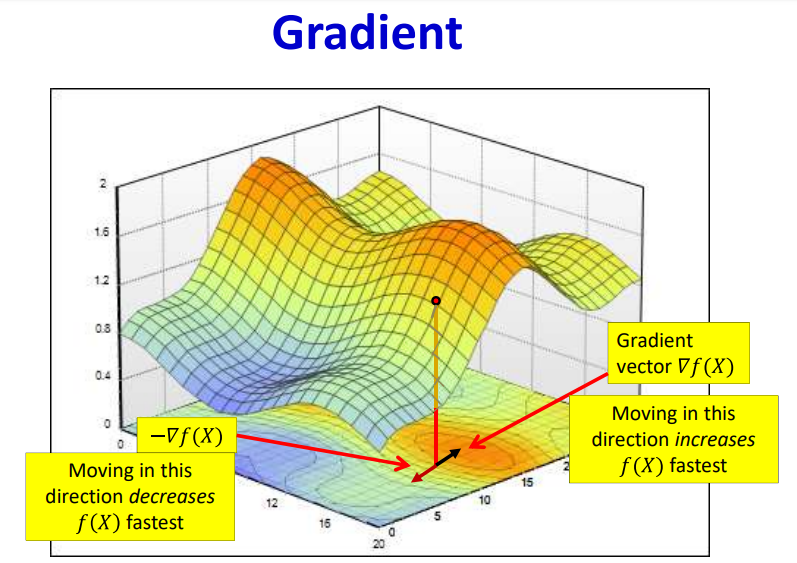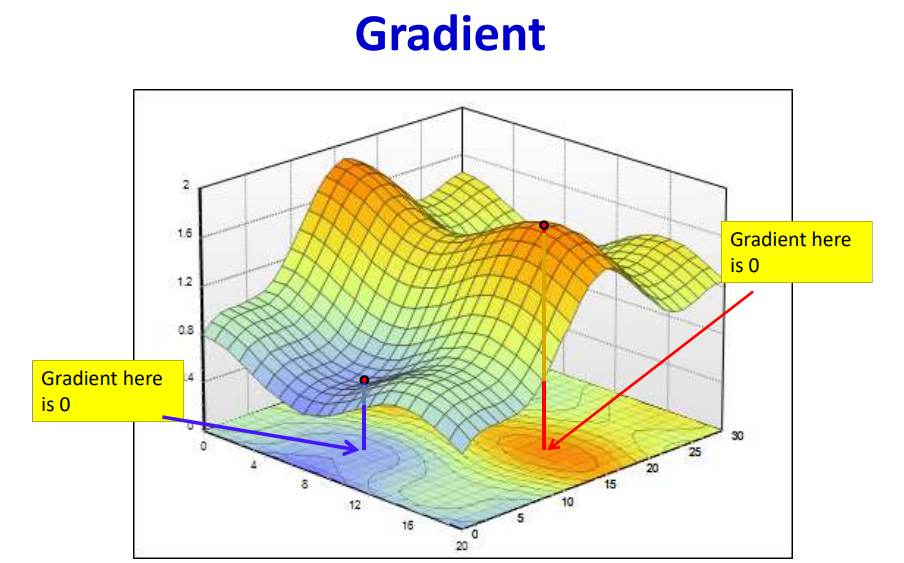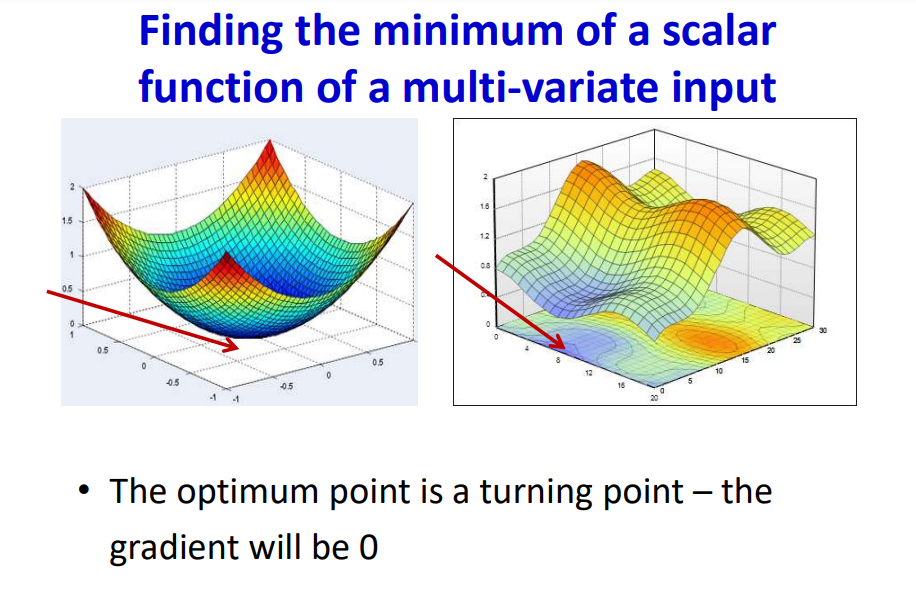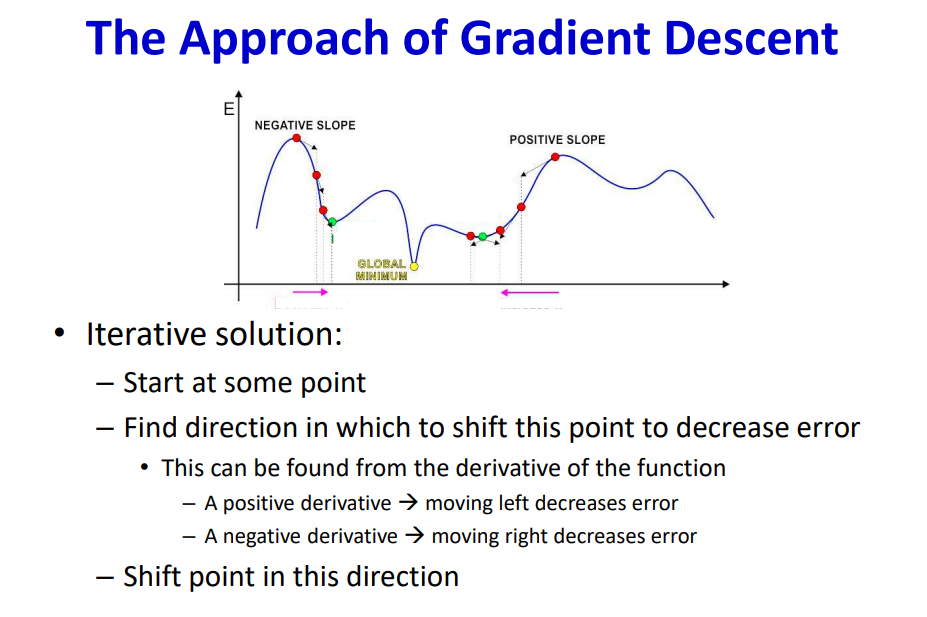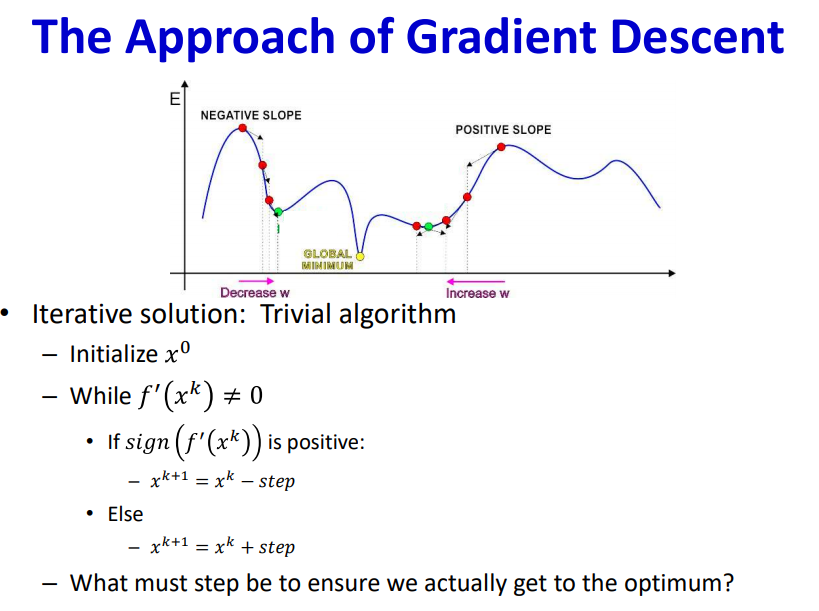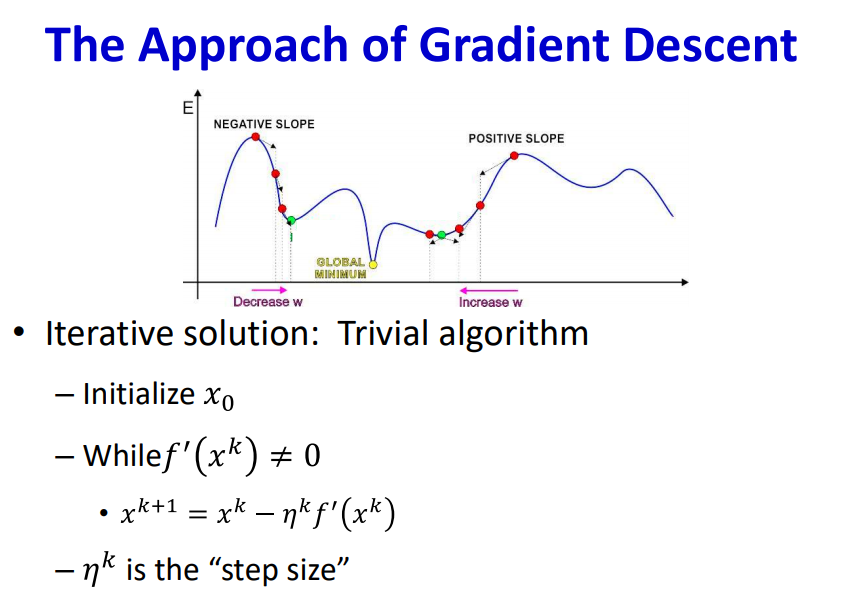The gradient as a multiple of step size acts as weight updating factor. When this factor becomes zero, this causes vanishing gradient problem. Vanishing gradient occurs when there is no weight update which lowers the loss function.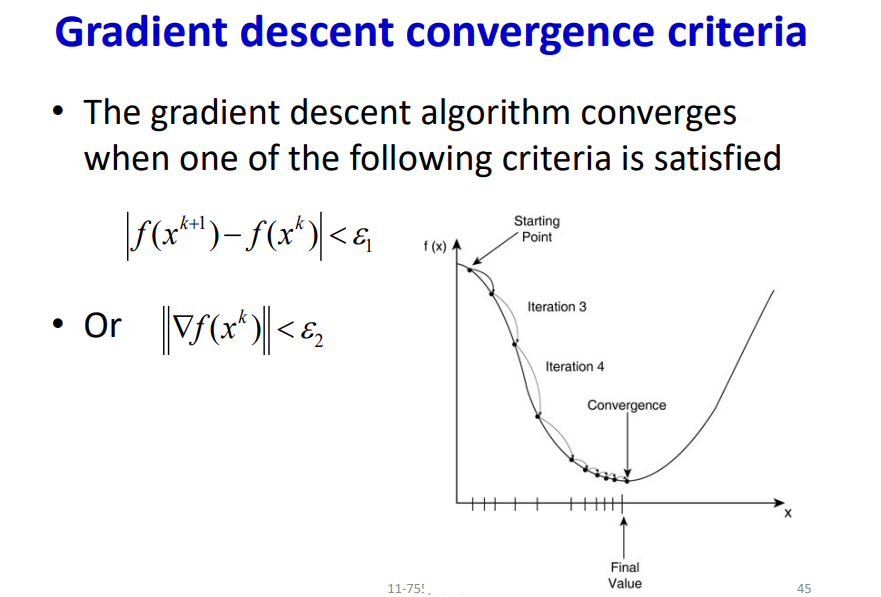• The neural network attains convergence when the difference in loss function is less than ε.

• ε depends on the minimum loss that can be attained from a given data distribution.